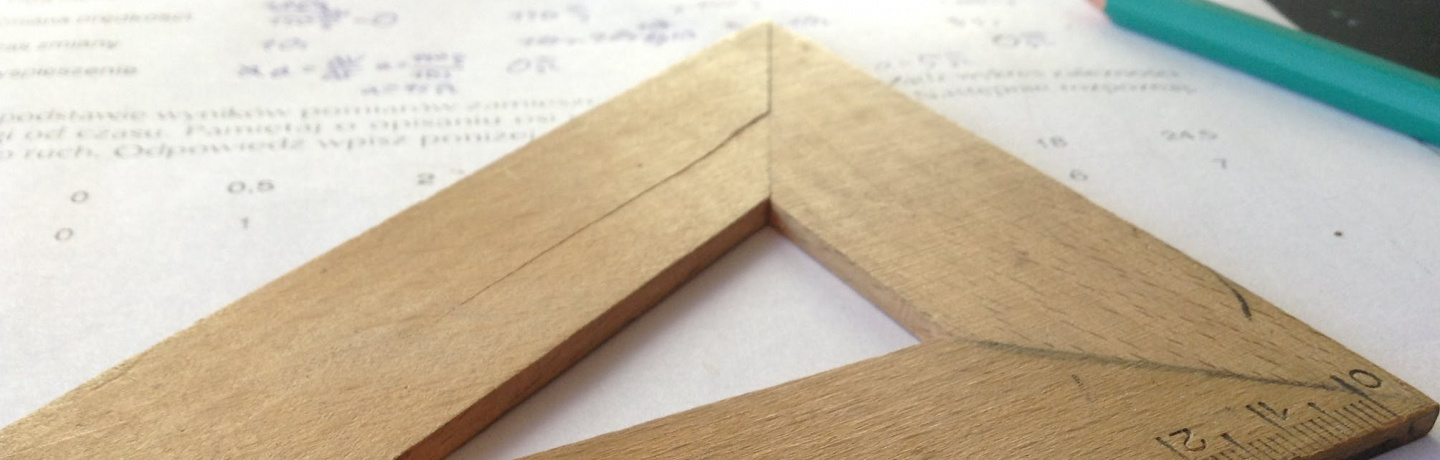# High-Order Numerical Methods for Fractional Ordinary Differential Equations# High-Order Numerical Methods for Fractional Ordinary Differential Equations

### —

Luís Ferrás

CMAT, University of Minho

Taking into account the regularity properties of the solutions of fractional differential equations, we develop a numerical method which is able to deal, with the same accuracy, with both smooth and nonsmooth solutions of systems of fractional ordinary differential equations of the Caputo-type. We provide the error analysis of the numerical method and we illustrate its feasibility and accuracy through some numerical examples. Finally, we solve the time-fractional diffusion equation using a combination of the method of lines and the newly developed hybrid method.